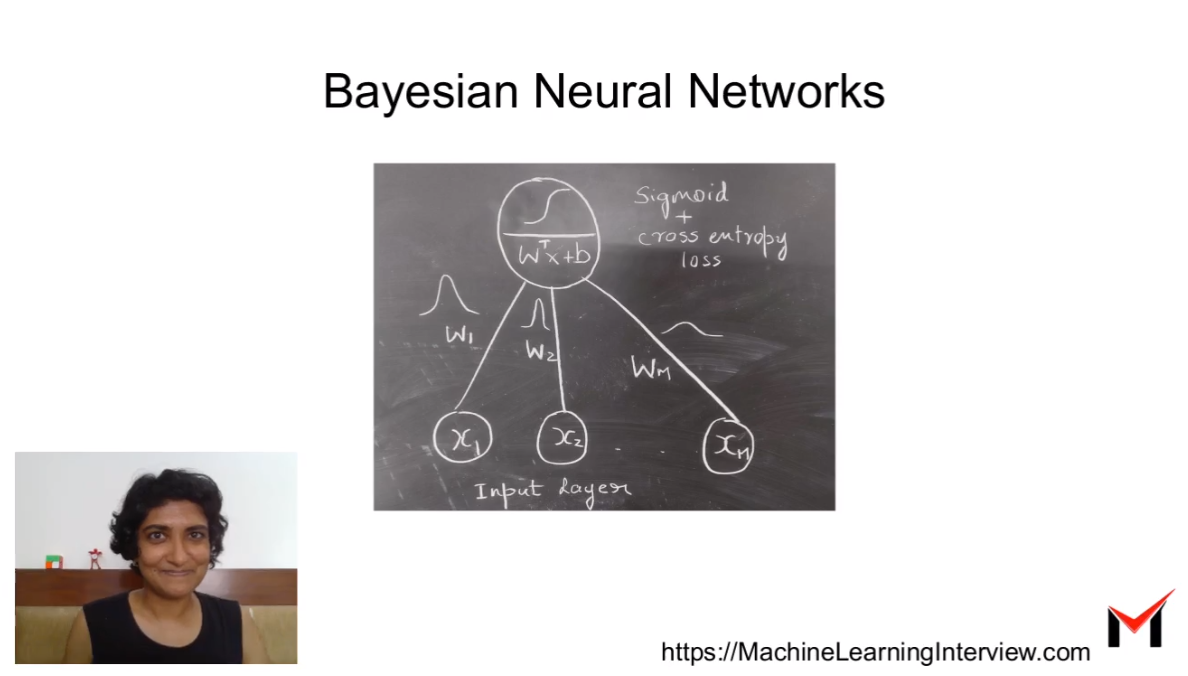# Bayesian Neural Networks

Bayesian Neural networks enable capturing uncertainity in the parameters of a neural network.

This video contains:

1. A brief Recap of Feedforward Neural Networks
2. Motivation behind a Bayesian Neural Network
3. What is a Bayesian Neural Network
4. Inference in a Bayesian Neural Network
5. Pros and Cons of using a Bayesian Neural Network
6. References to Code samples to get started with using Bayesian Neural Networks